# ML Wiki

## Motivation

• Suppose we have a large number of features and a complex structure of data
• For Logistic Regression we would probably need to fit a very high-order polynomial

Say we have 100 features ($n = 100$) and we want to fit, a multiplication of each pair of features

• i.e. we will have $x_1^2, x_1 x_2, ..., x_1 x_{100}, ... x_2^2, x_2 x_3, ...,$
• this gives us $\approx$ 5000 features (it grows as $O(n)$)
• for combinations of triples we'll have $\approx$ 170 000 features!

Next, suppose we have a computer vision problem: car detection

• we show it cars, then show it not cars, and then test
• Say we have 50 x 50 pixels image, 2500 pixels in total (7500 if RGB).
• If we want to fit polynomials, the number of features is too huge to do this!

So using Logistic Regression is certainly not a good way to handle lots of features, and here Neural Networks can help

## Neural Networks

This technique is based on how our brain works - it tries to mimic its behavior.

## Model Representation

• A NN model is built from many neurons - cells in the brain.
• the neuron, called activation unit, takes features as input
• A network consists of many activation units

### Sigmoid Activation Unit

it's called sigmoid (logistic) activation function: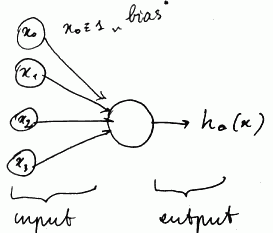Bias unit

• $x_0$ is a bias unit, $x_0 = 1$ always
• $x_0$ may be omitted from a picture, but it's usually assumed in these cases

Weights

• arrows are "input wires"
• so this unit takes $x = [x_0, x_1, x_2, x_3]^T$,
• and the wires are out parameters $\theta = [\theta_0, \theta_1, \theta_2, \theta_3]^T$ - their are called weights

Result

• it applies the sigmoid function to the input, and as the result, it returns
• $h_{\theta} = g(\theta^T x) = \cfrac{1}{1 + e^{-\theta^T x}}$

### Neural Network Model

Let's have a look at an actual neural network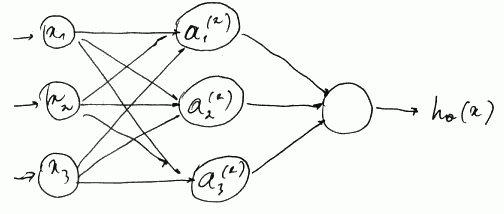• A NN model is a network of many sigmoid activation units, organized in layers
• where the next layer's input is the current layer's output
• the first layer is input layer, called $x$ - it takes our feature vector $x = [x_1, ..., x_n]^T$
• the last layer (3rd on the picture) is an output layer, it gives us the final result
• all layers in between are called hidden layers
• (note that bias units $x_0$ and $a_0^{(2)}$ are omitted from the picture, but they are there)

### Mathematical Representation

We'll have the following notation:

• $a_i^{(j)}$ is an activation of unit $i$ in layer $j$
• $\theta^{(j)}$ - matrix of weights that control mapping from layer $j$ to $j + 1$ (i.e. $\theta_1$ is the parameters of the 2nd layer and so on)
• Neural Networks are parametrized by $\theta$s

Mathematical representation of a neural network is (where $g$ is the sigmoid function)

• $a_1^{(2)} = g(\theta_{10}^{(1)} x_0 + \theta_{11}^{(1)} x_1 + \theta_{12}^{(1)} x_2 + \theta_{13}^{(1)} x_3)$
• $a_2^{(2)} = g(\theta_{20}^{(1)} x_0 + \theta_{21}^{(1)} x_1 + \theta_{22}^{(1)} x_2 + \theta_{23}^{(1)} x_3)$
• $a_3^{(2)} = g(\theta_{30}^{(1)} x_0 + \theta_{31}^{(1)} x_1 + \theta_{32}^{(1)} x_2 + \theta_{33}^{(1)} x_3)$
• and $h_{\theta}(x) = a_1^{(3)} = g(\theta_{10}^{(2)} a_0^{(2)} + \theta_{11}^{(2)} a_1^{(2)} + \theta_{12}^{(2)} a_2^{(2)} + \theta_{13}^{(2)} a_3^{(2)})$

so we have

• 3 input units $x_1, x_2, x_3$ (plus bias $x_0 = 1$)
• 3 hidden units in 1 hidden layer $a_1^{(2)}, a_2^{(2)}, a_3^{(2)}$ in layer 2 (plus bias $a_0^{(2)} = 1$)

### Dimension of $\theta$

In this example the dimension of $\theta^{(1)}$ is $\theta^{(1)} \in \mathbb{R}^{3 \times 4}$

General rule

• if a network has $s_j$ units in layer $j$ and $s_{j + 1}$ units in layer $j + 1$ , then
• $\theta^{(i)} \in \mathbb{R}^{s_{j + 1} \times (s_{j} + 1)}$ (i.e. it has dimension $s_{j + 1} \times (s_{j} + 1)$)

## Forward Propagation

### Vectorized Form

For the first step we have

• $a_1^{(2)} = g(z_1^{(2)})$ where $z_1^{(2)} = \theta_{10}^{(1)} x_0 + \theta_{11}^{(1)} x_1 + \theta_{12}^{(1)} x_2 + \theta_{13}^{(1)} x_3$
• $a_2^{(2)} = g(z_2^{(2)})$, and
• $a_3^{(2)} = g(z_3^{(2)})$
• ($z_1^{(2)}, z_2^{(2)}, z_3^{(2)}$ - are linear combinations of $x_1, x_2, x_3$)

So we have 3 vectors

• $\theta^{(0)} = [\theta_0^{(0)}, \theta_1^{(0)}, \theta_2^{(0)}, \theta_3^{(0)}]^T$,
• $x = [x_0, x_1, x_2, x_3]^T$,
• $z^{(2)} = [z_1^{(2)}, z_2^{(2)}, z_3^{(2)}]^T$

And can rewrite the first step in a vectorized form:

• instead of $z_1^{(2)} = \theta_{10}^{(1)} x_0 + \theta_{11}^{(1)} x_1 + \theta_{12}^{(1)} x_2 + \theta_{13}^{(1)} x_3$, we write $z_1^{(2)} = \theta_1^{(1)} \cdot x$

### Algorithm

• 1st step
• $z^{(2)} = \theta^{(1)} \cdot x = \theta^{(1)} \cdot a^{(1)}$ and
• $a^{(2)} = g(z^{(2)}) \in \mathbb{R}^3$
• next step
• we add $a_0^{(2)} = 1$, so $a^{(2)} = [1, a_1^{(2)}, a_2^{(2)}, a_3^{(2)}]^T \in \mathbb{R}^{4}$
• and calculate $z^{(3)} = \theta^{(2)} \cdot a^{(2)}$
• finally
• $h_{\theta}(x) = a^{(3)} = g(z^{(3)})$

This process is called forward propagation

### What's going on?

Let's have a look at the 2nd and 3rd layers of our NN• $h_{\theta} = g((\theta^{(2)})^T \cdot a^{(2)})$, and $a^{(2)}$ is given by the 2nd level units
• so it's doing a logistic regression, but it uses $a^{(2)} = [a_0^{(2)} ... a_3^{(2)}]$ for features (instead of $x$s)
• and features $a^{(2)} = [a_0^{(2)} ... a_3^{(2)}]$ are themselves learned by the previous layer

• We can create NNs with as many layers as we want
• The way the neurons are connected is called architecture

## Multi-class Classification

What to do if we what to use it for multi-class classification?

So we want

• $h_{\theta}(x) \approx \left[\begin{matrix} 1 \\ 0 \\ 0 \\ 0\end{matrix} \right]$ if an item belongs to 1st category
• $h_{\theta}(x) \approx \left[\begin{matrix} 0 \\ 1 \\ 0 \\ 0\end{matrix} \right]$ if it belongs to 2nd category
• and so on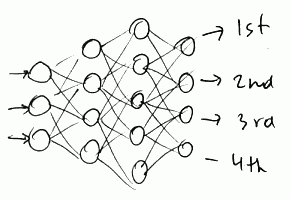For training set ${(x^{(i)}, y^{(i)})}$

• we turn $y$ into one of $\left\{ \left[\begin{matrix} 1 \\ 0 \\ 0 \\ 0\end{matrix} \right], \left[\begin{matrix} 0 \\ 1 \\ 0 \\ 0\end{matrix} \right], \left[\begin{matrix} 0 \\ 0 \\ 1 \\ 0\end{matrix} \right], \left[\begin{matrix} 0 \\ 0 \\ 0 \\ 1\end{matrix} \right] \right\}$ - instead of $y \in \{1, 2, 3, 4\}$,
• so, when training, we would like to have $h_{\theta}(x^{(i)}) \approx y^{(i)} \in \mathbb{R}^4$
• then we select the class with highest $h_{\theta}^{(i)}(x^{(i)})$, as in One-vs-All Classification

## Cost Function

Suppose we have $m$ training examples $\{(x^{(i)}, y^{(i)})\}$

• $L$ - total number of layers in network
• $S_l$ - # of units (without bias) in layer $l$
• $K = S_L$ - number of units the output layer, i.e. the network classifies $K$ classes

output $h_{\theta}(x) \in \mathbb{R}^{K}$

For example,

•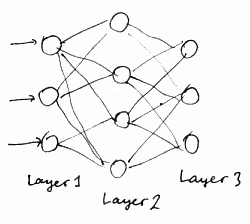• $L = 3$
• $S_1 = 3, S_2 = 4, K = S_3 = 3$

For Logistic Regression with Regularization we have the following cost function:

$$J(\theta) = -\cfrac{1}{m} \sum \Big[ y^{(i)} \log h_{\theta}(x^{(i)}) + (1 - y^{(i)}) \log (1 - h_{\theta}(x^{(i)})) \Big] + \cfrac{\lambda}{2m} \sum_{j = 1}^{n} \theta_j^2$$

In neural networks

• $h_{\theta}(x) \in \mathbb{R}^K$
• let $h_{\theta}(x)_i$ - be $i$th output

So we have

$$J(\theta) = -\cfrac{1}{m} \sum_{i = 1}^{m} \sum_{k = 1}^{K} \Big[ y_k^{(i)} \log h_{\theta}(x^{(i)})_k + (1 - y_k^{(i)}) \log (1 - h_{\theta}(x^{(i)})_k ) \Big] + \cfrac{\lambda}{2m} \sum_{l = 1}^{L - 1} \sum_{i = 1}^{S_l} \sum_{j = 1}^{S_{l + 1}} (\theta_{ji}^{l})^2$$

here we also don't regularize bias inputs

## Back Propagation

• we need to find such $\theta$ that $J(\theta)$ is minimal
• for that we can use Gradient Descent or other advanced optimization techniques
• for GD we need to compute partial derivative $\cfrac{\partial}{\partial \theta_{ij}^{(l)}} J(\theta)$ with respect to each $\theta_{ij}^{(l)}$

Back Propagation is a technique for calculating partial derivatives in neural networks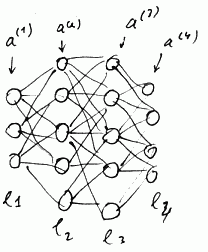suppose we have a training example $(x, y)$

To compute cost $J(\theta)$ we use Forward Propagation (vectorized)
• $a^{(1)} = x$
• $z^{(2)} = \theta^{(1)} \cdot a^{(1)}$
• $a^{(2)} = g(z^{(2)})$ (plus adding $a_0^{(2)} = 1$)
• $z^{(3)} = \theta^{(2)} \cdot a^{(2)}$
• $a^{(3)} = g(z^{(3)})$ (plus adding $a_0^{(3)} = 1$)
• $z^{(4)} = \theta^{(3)} \cdot a^{(4)}$
• $a^{(4)} = g(z^{(4)})$ (plus adding $a_0^{4)} = 1$)

### Back Propagation Overview

To compute derivatives we use Back Propagation

• let $\delta_j^{(l)}$be "error" of node $j$ in layer $i$ (for $a_j^{(l)}$)
• for each output unit we compute
• $\delta_k^{(L)} = a_j^{(L)} - y_j = h_{\theta}(x)_j - y_j$
• or, vectorized:
$\delta^{(L)} = a^{(L)} - y$

next, we compute $\delta$ for all earlier layers

• $\delta^{(3)} = (\theta^{(3)})^T \cdot \delta^{(4)} * g'(z^{(3)})$
• where
• $*$ - element-wise product
• $g'(z^{(3)})$ - derivative of function $g$ in $z^{(3)}$
• $g'(z^{(3)}) = a^{(3)} * (1 - a^{(3)})$
• $\delta^{(2)} = (\theta^{(2)})T \cdot \delta^{(3)} .* g'(z^{(2)})$
• We don't compute anything for the first layer - it's the input layer, and there can be no errors

Our partial derivative is

• $\cfrac{\partial}{\partial \theta_{ij}^{(l)}} J(\theta) = a_j^{(l)} \cdot \delta_i^{(l + 1)}$
• (here we ignore regularization parameter $\lambda$ - i.e. we assume no regularization at the moment)

### Back Propagation Algorithm

We have training set $\{(x^{(i)}, y^{(i)}\}$ with $m$ training examples

Set $\Delta_{ij}^{(l)} \leftarrow 0$ for all $l$, $i$, $j$ (it's used to compute$\cfrac{\partial}{\partial \theta_{ij}^{(l)}} J(\theta)$ )

For each $\{(x^{(i)}, y^{(i)}\}$:

• set $a^{(1)} = x^{(i)}$
• perform Forward Propagation to compute $a^{(l)}$ for $l = 2, 3, ..., L$
• perform Back Propagation
• Using $y^{(i)}$ compute $\delta^{(L)} = a^{(L)} - y^{(i)}$
• Then compute
• all $\delta^{(L - 1)}, \delta^{(L - 2)}, ..., \delta^{(2)}$
• Set $\Delta_{ij}^{(l)} \leftarrow \Delta_{ij}^{(l)} + a_j^{(l)} \delta_i^{(l + 1)}$
• or, vectorized:
$\Delta^{(l)} \leftarrow \Delta^{(l)} + \delta^{(l + 1)} (a^{(l)})^T$
• Then we calculate
• $D_{ij}^{(l)} \leftarrow \cfrac{1}{m} \delta_{ij}^{(l)} + \lambda \theta_{ij}^{(l)}$ if $j \ne 0$
• $D_{ij}^{(l)} \leftarrow \cfrac{1}{m} \delta_{ij}^{(l)}$ if $j = 0$
• That value can we used for GD:
$\cfrac{\partial}{\partial \theta_{ij}^{(l)}} = D_{ij}^{(l)}$

To sum up, for each training example we

• propagate forward using $x$
• we back-propagate using $y$
• add up all error units (for each $\theta$) into matrix $\Delta$

### Intuition

Say we have a single training example $(x, y)$ and 1 output unit and we ignore regularization

$\text{cost}(x, y) = y \log h_{\theta}(x) + (1 - y) \log h_{\theta}(x)$

• it calculates how well is the network doing for that training example - how close it is to $y$?
• $\delta_j^{(l)}$ = "error" of cost for $a_j^{(l)}$ (unit $j$ in layer $l$)

formally,

• $\delta_j^{(l)} = \cfrac{\partial}{\partial z_j^{(l)}} \text{cost}(x, y)$
• it's a partial derivative with respect to $z_j^{(l)}$
• they are measures how much we would like to change the NN weights in order
• to affect intermediate values $z^{(2)}, z^{(3)}, ...,$
• and the final output $z^{(4)}$,
• and therefore, overall cost

### Numerical Gradient Checking

Implementing back-propagation can be hard and bug-prone, but we can perform Numerical Gradient Checking to test our implementation

suppose $\theta \in \mathbb{R}$

• We can estimate real slope of the derivative by calculating $J(\theta + \epsilon) - J(\theta - \epsilon)$ for small $\epsilon$
•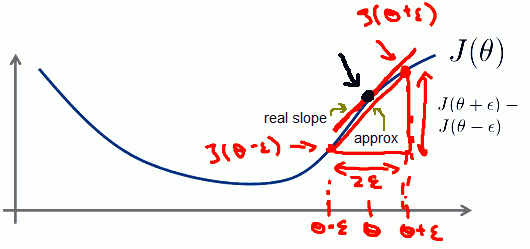• $\cfrac{d}{d \theta} J(\theta) \approx \cfrac{J(\theta + \epsilon) - J(\theta - \epsilon)}{2\epsilon}$
• And that will give us a numerical estimate of the gradient and that point

when $\theta \in \mathbb{R}^n$

• for partial derivative with respect to each $\theta_i$ we calculate
• $\cfrac{\partial}{\partial \theta_1} J(\theta) \approx \cfrac{J(\theta_1 + \epsilon, \theta_2, ..., \theta_n) - J(\theta_1 - \epsilon, \theta_2, ..., \theta_n)}{2\epsilon}$
• $\cfrac{\partial}{\partial \theta_2} J(\theta) \approx \cfrac{J(\theta_1, \theta_2 + \epsilon, ..., \theta_n) - J(\theta_1, \theta_2 - \epsilon, ..., \theta_n)}{2\epsilon}$
• ...
• $\cfrac{\partial}{\partial \theta_2} J(\theta) \approx \cfrac{J(\theta_1, \theta_2, ..., \theta_n + \epsilon) - J(\theta_1, \theta_2, ..., \theta_n - \epsilon)}{2\epsilon}$
• i.e. we add and subtract only values for $\theta_i$ we calculate derivative for
• this gives us a way to numerically estimate all partial derivatives
• and then we check if this numerical estimate $\approx$ the derivative from back propagation

### Implementation Notes

To implement Back Propagation use the following approach:

• Implement back propagation to compute $D^{(1)}, D^{(2)}, ...$
• Implement numerical gradient check to compute approximations of partial derivatives
• Make sure they have similar values
• Turn off gradient checking, use only back propagation for learning (it's much more computationally efficient)

## Random Initialization

• We need to have initial values for $\theta$
• In Logistic Regression we used $\theta = [0, 0, ..., 0]^T$
• It won't work for NNs

Suppose we set all $\theta_{ij}^{(l)}$ to 0

• Then we'll have same values for $a_1^{(2)} = a_2^{(2)} = ... = a_{s_2}^{(2)}$ and same for $\delta^{(2)}$
(after each update, parameters corresponding to inputs are identical, and all hidden units will compute the same value)
• And therefore, all partial derivatives will also we equal
• This is called the problem of symmetric weights

We can break the symmetry with random initialization

• so, initialize each $\theta_{ij}^{(l)}$ with random value from $[-\epsilon; \epsilon]$:
• $\theta_{ij}^{(l)} \leftarrow_{r} [-\epsilon; \epsilon]$

## Implementation

### Algorithm

NB: $J(\theta)$ in non-convex and can get stuck in local minimum - but usually it's not a problem